# 1 Goal

## 1.1 Party trick

A fun party trick is to take vector and order it using order()

x <- runif(5)
x
##  0.7809753 0.5210077 0.8932159 0.5110514 0.2235770
xo <- x[order(x)]
xo
##  0.2235770 0.5110514 0.5210077 0.7809753 0.8932159

The original order can the be retrieved by applying order() twice, and using the result to order xo

xo[order(order(x))]
##  0.7809753 0.5210077 0.8932159 0.5110514 0.2235770

Neat, eh? The ordered vector xo can be transformed in a way that might be difficult for the original data, for instance finding differences between ordered values

diff(c(0, xo))[order(order(x))]
##  0.259967575 0.009956273 0.112240639 0.287474455 0.223576978

or transforming numeric values into letters, such that the smallest value of x is given the first letter, etc.

LETTERS[seq_along(xo)][order(order(x))]
##  "D" "C" "E" "B" "A"

## 1.2 Quantile normalization

Create a matrix with two columns representing ‘gene expression’ of 100 (actually, 10,000’s of) genes in two samples. The idea is that ‘base-line’ expression follows some kind of exponential distributition

set.seed(123)
n_genes <- 1000
base <- 2 + rexp(n_genes)

and that the expression observed in each sample is the baseline plus some (normally distributed) error. The following assumes that the size of the measurement error decreases as the gene expression increases; sample y has (e.g., because of sample processing) a consistently stronger signal than sample x.

expression <- data.frame(
x = base + rnorm(n_genes, 0.3) / base,
y = 1.1 * base + rnorm(n_genes, 0.4) / base
)

Visualize the expression of each gene in the two samples…

library(ggplot2)
ggplot(expression, aes(x, y)) + geom_point()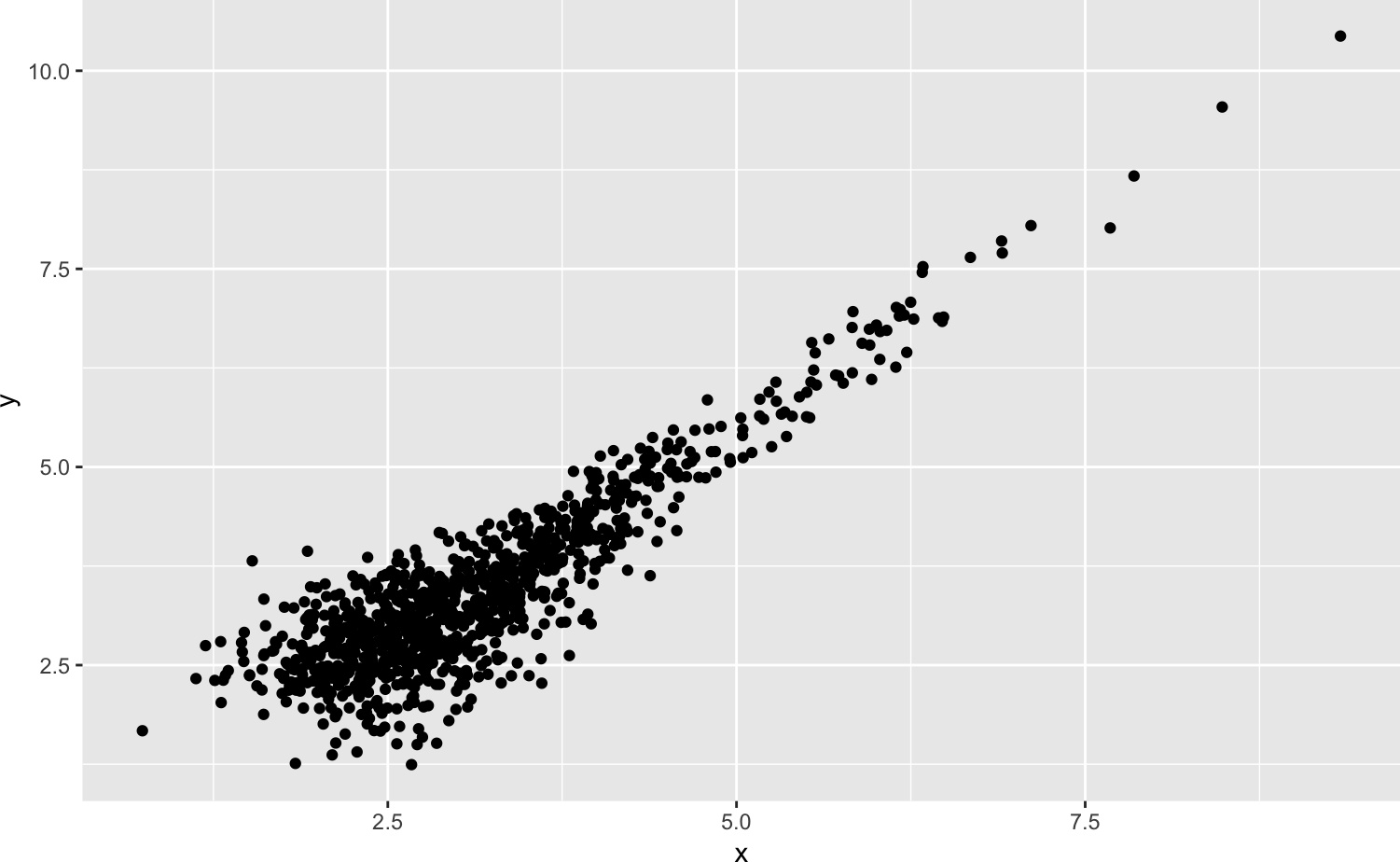and the distribution of expression values across all genes

df <- reshape::melt(expression)
## Using  as id variables
ggplot(df, aes(x = value, colour = factor(variable))) +
geom_density()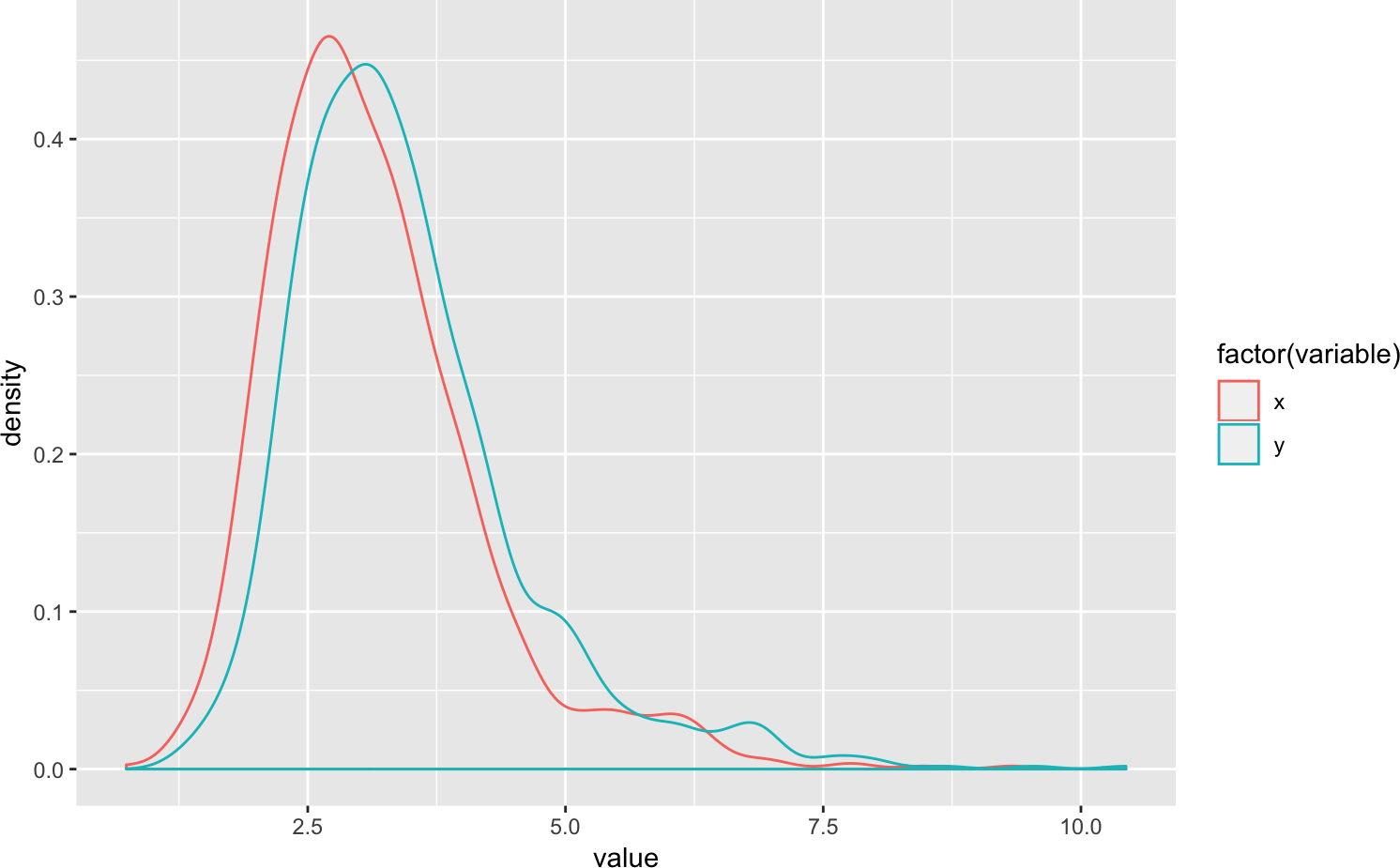A commmon hypothesis in, e.g., RNA-seq, is that the expression of most genes is unchanged, and differences between distributions are due to technical artifacts. Under this assumption, we expect the distributions in the second figure, above, to be identical. So we could try to normalize samples so that the distributions of each sample look about the same. Quantile normalization is an extreme form of this normalization, and makes the distributions exactly the same.

1. Place each column in order

x <- expression$x xo <- x[order(x)] y <- expression$y
yo <- y[order(y)]

For our sample of 100, x is the first quantile, x the second, etc.

2. Calculate the mean (or more robust, e.g., median) statistic for each quantile. I’ll do this by using cbind() to create a two-column matrix, and then apply() to iterate over each row to calculate the mean

mo <- cbind(xo, yo)
row_mean <- apply(mo, 1, mean)
3. Use the mean of each quantile in the original vector

normalized <- data.frame(
x = row_mean[order(order(x))],
y = row_mean[order(order(y))]
)

Visualize normalized data – same overall relationship between expression in two samples

ggplot(normalized, aes(x, y)) + geom_point()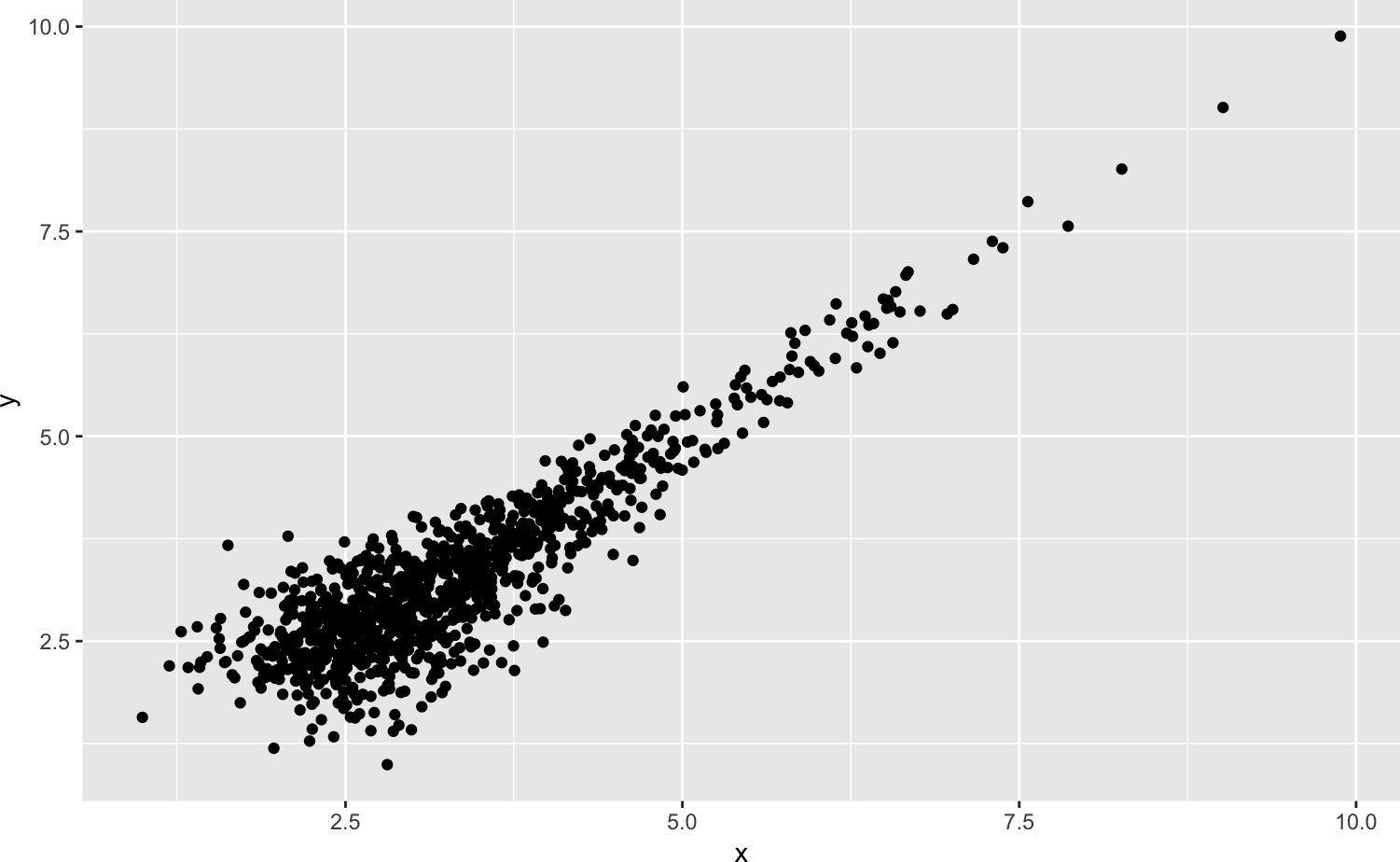…but now identical distributions

df <- reshape::melt(normalized)
## Using  as id variables
ggplot(df, aes(x = value, colour = factor(variable))) +
geom_density()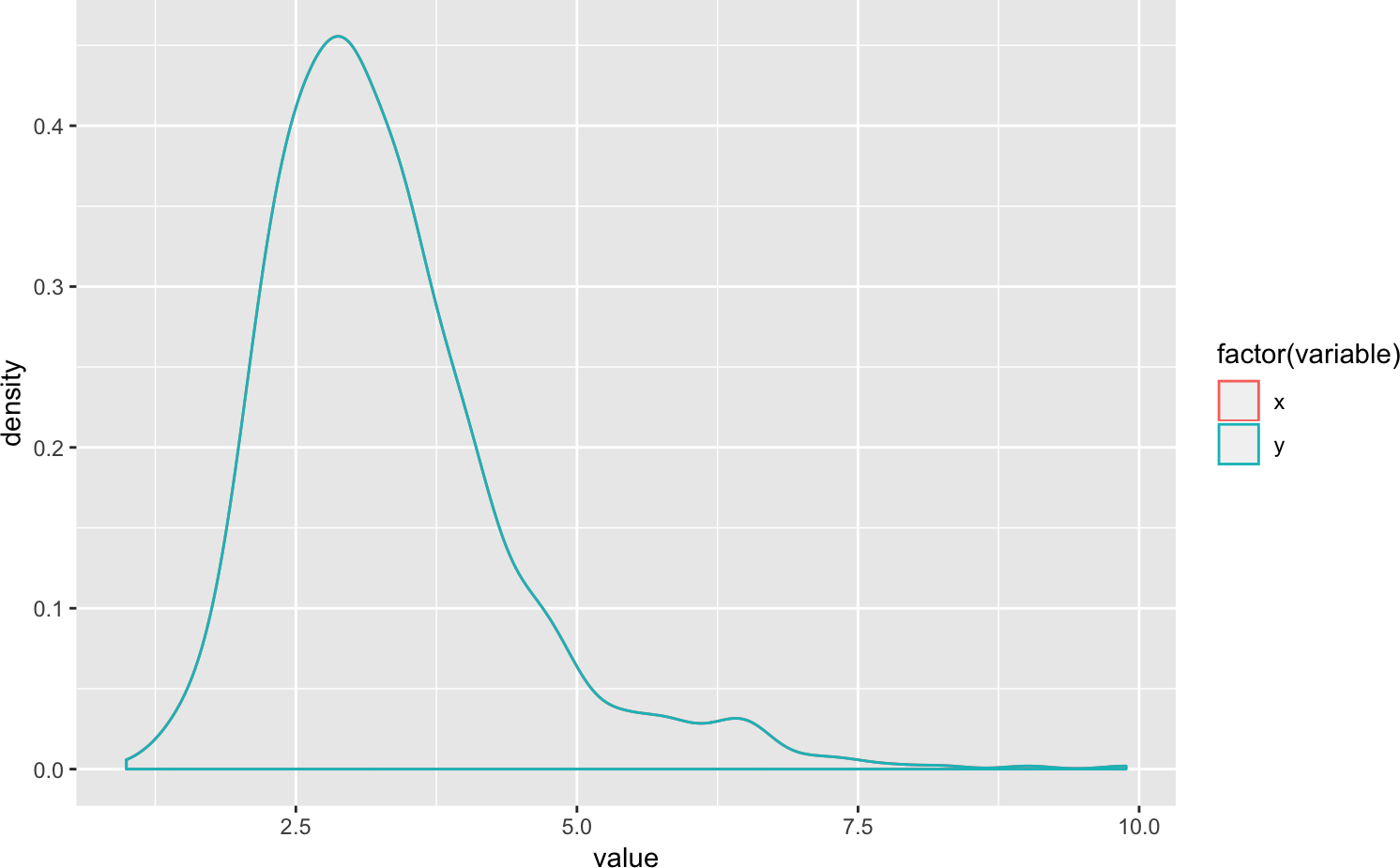# 2 From one-off script to reusable function

## 2.1 Functions

We could write a script, copy/pasting each time we wanted to use this

x <- expression$x xo <- x[order(x)] y <- expression$y
yo <- y[order(y)]
mo <- cbind(xo, yo)
row_mean <- apply(mo, 1, mean)
normalized <- data.frame(
x = row_mean[order(order(x))],
y = row_mean[order(order(y))]
)

It would be better to write a function

quantile_normalize <-
function(expression)
{
x <- expression$x xo <- x[order(x)] y <- expression$y
yo <- y[order(y)]

mo <- cbind(xo, yo)
row_mean <- apply(mo, 1, mean)

data.frame(
x = row_mean[order(order(x))],
y = row_mean[order(order(y))]
)
}

and use that on different data sets.

normalized <- quantile_normalize(expression)

## 2.2 Testing

We’re going to update our function to be more efficient, general, and robust, but we want to have some confidence that the function still works as expected. So we’ll make a much smaller data set that we can verify ‘by hand’ and use as a test case.

expression <- data.frame(
x = c(1, 2, 4, 7),
y = c(3, 2, 5, 8)
)
quantile_normalize(expression)
##     x   y
## 1 1.5 2.5
## 2 2.5 1.5
## 3 4.5 4.5
## 4 7.5 7.5

We can use a formal concept called a ‘unit test’ to check that our code is correct

library(testthat)
test_that("quantile_normalize() works", {
## calculate simple example 'by hand'...
x <- c(1, 2, 4, 7)
y <- c(3, 2, 5, 8)
xo <- x[order(x)]
yo <- y[order(y)]
mo <- apply(cbind(xo, yo), 1, mean)
expected <- data.frame(x = mo[order(order(x))], y = mo[order(order(y))])

## Compare to outcome of our function
expression <- data.frame(x = x, y = y)
observed <- quantile_normalize(expression)
expect_equal(observed, expected)      # other expectations possible
})

# 3 A little more…

## 3.1 General

Our function assumes that there are exactly two samples, and that the two samples are labelled x and y. Let’s try and generalize our function using apply() to order an arbitrary number of columns,

m <- apply(expression, 2, function(v) v[order(v)])
m
##      x y
## [1,] 1 2
## [2,] 2 3
## [3,] 4 5
## [4,] 7 8

and apply the same sort of logic to reconstruct the quantiles

quantile_normalize <-
function(x)
{
m <- apply(x, 2, function(v) v[order(v)])
row_mean <- apply(m, 1, mean)
apply(x, 2, function(v, row_mean) row_mean[order(order(v))], row_mean)
}

Our unit test will fail, because the original function returned a data.frame, whereas the current function returns a matrix. Let’s update the unit test (we could also coerce the matrix to a data.frame before returning from quantile_normalize()).

test_that("quantile_normalize() works", {
x <- c(1, 2, 4, 7)
y <- c(3, 2, 5, 8)
xo <- x[order(x)]
yo <- y[order(y)]
mo <- apply(cbind(xo, yo), 1, mean)
expected <- cbind(x = mo[order(order(x))], y = mo[order(order(y))])

expression <- data.frame(x = x, y = y)
observed <- quantile_normalize(expression)
expect_equal(observed, expected)
})

## 3.2 Efficient

apply() is an iteration – the function is called once for each element of m. We could instead use rowMeans(), which is vectorized and makes only one function call for the entire data. This is more efficient in R, so let’s try to modify our function

quantile_normalize <-
function(x)
{
m <- apply(x, 2, function(v) v[order(v)])
row_mean <- rowMeans(m)
apply(x, 2, function(v, row_mean) row_mean[order(order(v))], row_mean)
}

Our unit test still works

test_that("quantile_normalize() works", {
x <- c(1, 2, 4, 7)
y <- c(3, 2, 5, 8)
xo <- x[order(x)]
yo <- y[order(y)]
mo <- apply(cbind(xo, yo), 1, mean)
expected <- cbind(x = mo[order(order(x))], y = mo[order(order(y))])

expression <- data.frame(x = x, y = y)
observed <- quantile_normalize(expression)
expect_equal(observed, expected)
})

so we have some confidence that we’ve changed our implementation without changing the outcome.

## 3.3 Robust

Good functions provide something like a contract – if certain types of arguments are provided, then the function will return a certain type of value. In our case we might think of the contract as “if you provide me with a data.frame with all numeric columns, or a numeric matrix, I’ll provide you with a matrix of quantile-normalized columns”. There could be additional constraints on the contract, for instance “the input cannot have NA values” or “dimnames of the input are the same as dimnames of the output”. Checking that the inputs satisfy the contract greatly simplifies our function, and typically provides the user with helpful indications before things go wrong in a cryptic way. So it seems like we should validate the inputs of the function as soon as possible.

quantile_normalize <-
function(x)
{
## so long as the input can be coerced to a matrix...
x <- as.matrix(x)

## and can be validated to conform to our contract...
stopifnot(
is.numeric(x),
!anyNA(x)
)

## ...we have confidence that we will satisfy the return value
m <- apply(x, 2, function(v) v[order(v)])
row_mean <- rowMeans(m)
apply(x, 2, function(v, mo) mo[order(order(v))], mo)
}

We can check that our original unit test is satisfied, but also add aditional tests…

test_that("'quantile_normalize()' validates inputs", {
m <- cbind(letters, LETTERS)
expect_error(quantile_normalize(m))

df <- data.frame(x=rnorm(26), y = letters)
expect_error(quantile_normalize(df))

m <- matrix(rnorm(10), nrow = 5)
m[1,1] <- NA
expect_error(quantile_normalize(m))
})

It seems like our function should preserve dimnames on the original object. The function requires modification, and we can write a new unit test

quantile_normalize <-
function(x)
{
## validate inputs
x <- as.matrix(x)
stopifnot(
is.numeric(x),
!anyNA(x)
)

## quantile normalization
m <- apply(x, 2, function(v) v[order(v)])
row_mean <- rowMeans(m)
result <-
apply(x, 2, function(v, row_mean) row_mean[order(order(v))], row_mean)

## propagate dimnames
dimnames(result) <- dimnames(x)
result
}
test_that("'quantile_normalize()' propagates dimnames", {
m <- matrix(rnorm(10), 5, dimnames=list(LETTERS[1:5], letters[1:2]))
observed <- quantile_normalize(m)
expect_identical(dimnames(observed), dimnames(m))
})

We can also check that our function is robust to less common cases, like inputs with 0 rows and / or 0 columns. These tests lead to additional modifications – apply() does not always return a matrix!

quantile_normalize <-
function(x)
{
## validate inputs
x <- as.matrix(x)
stopifnot(
is.numeric(x),
!anyNA(x)
)

## quantile normalize
m <- apply(x, 2, function(v) v[order(v)])
dim(m) <- dim(x)    # apply() doesn't always return a matrix!

row_mean <- rowMeans(m)

result <- apply(
x, 2, function(v, row_mean) row_mean[order(order(v))], row_mean
)
dim(result) <- dim(x)

## propagate dimnames
dimnames(result) <- dimnames(x)
result
}
test_that("'quantile_normalize()' works with edge cases", {
m <- matrix(rnorm(5), nrow = 5)
expect_identical(m, quantile_normalize(m))

m <- matrix(rnorm(5), ncol = 5)
expect_identical(matrix(mean(m), ncol = 5), quantile_normalize(m))

m <- matrix(0, 0, 0)
expect_identical(m, quantile_normalize(m))
})

# 4 Performance

We’d like to get a sense of how our algorithm performs for various sized problems. We could use system.time() to evalute expressions, but a slightly more sophisticated approach replicates each timing to average over differences unrelated to our algorithm.

library(microbenchmark)

n_genes <- 10000
g10 <- matrix(rnorm(n_genes * 10), ncol = 10)
g100 <- matrix(rnorm(n_genes * 100), ncol = 100)
g1000 <- matrix(rnorm(n_genes * 1000), ncol = 1000)
g10000 <- matrix(rnorm(n_genes * 10000), ncol = 10000)

times <- microbenchmark(
quantile_normalize(g10),
quantile_normalize(g100),
quantile_normalize(g1000),
quantile_normalize(g10000),
times = 10
)

times
## Unit: milliseconds
##                        expr         min          lq        mean      median
##     quantile_normalize(g10)    13.62116    16.41978    35.16609    20.94962
##    quantile_normalize(g100)   141.18307   158.67358   209.78565   167.93833
##   quantile_normalize(g1000)  1443.09392  1620.43055  2649.53222  2817.20918
##  quantile_normalize(g10000) 18012.71678 20354.24486 22825.75161 21764.57106
##           uq        max neval
##     26.83651   138.9633    10
##    241.73374   394.6181    10
##   3298.01893  4772.0140    10
##  26625.01458 27220.2120    10
plot(times, log = "y")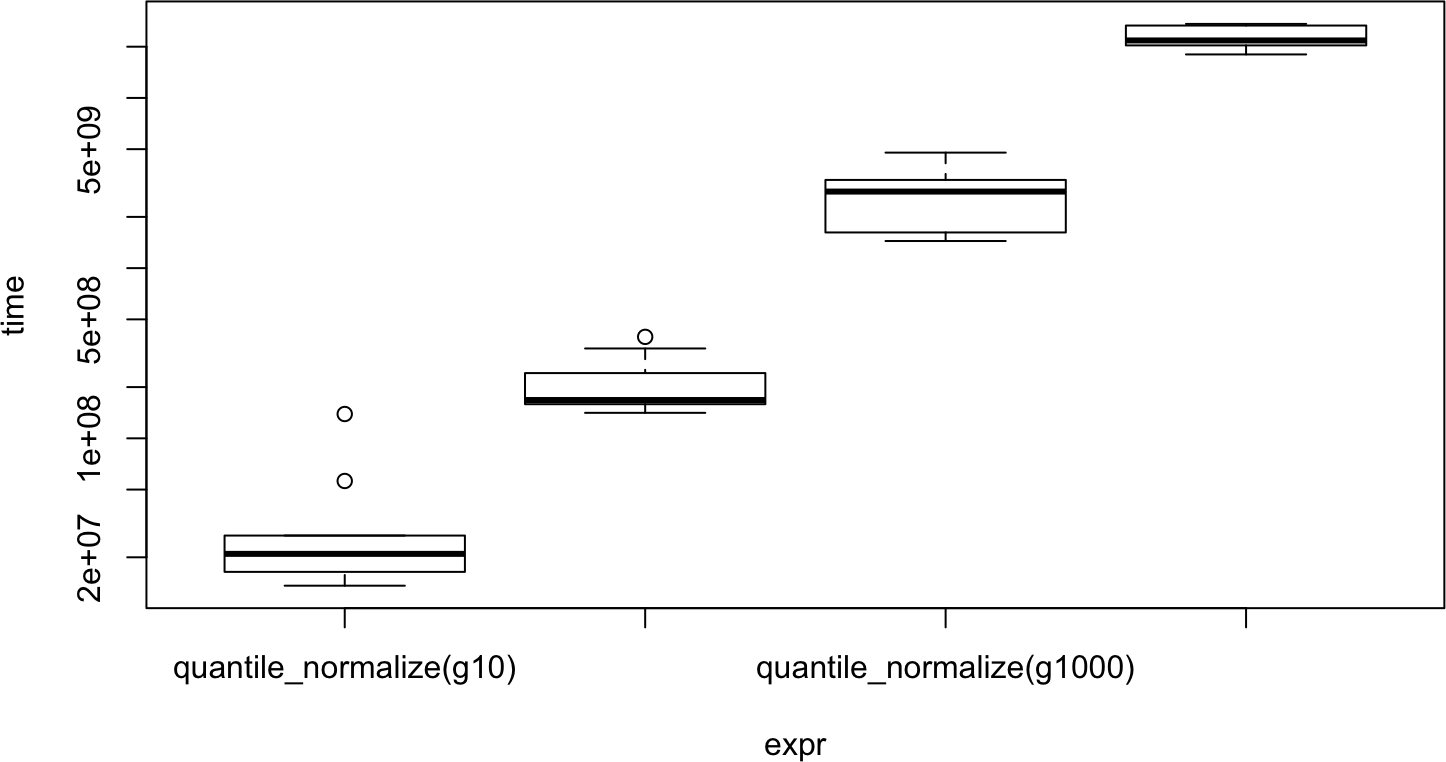The results show that the time to execute the algoritm scales linearly with the number of samples. Even 1000 samples takes only 3.5 seconds to normalize. Our implementation is ‘fast enough’ for many purposes.

Rprof() can be used to see where our alorithm spends its time

Rprof()        # start profiling
result <- quantile_normalize(g10000)
Rprof(NULL)    # stop profiling
summaryRprof()\$by.total

The output looks like

                     total.time total.pct self.time self.pct
"quantile_normalize"      32.30    100.00      0.00     0.00
"apply"                   31.92     98.82      2.92     9.04
"FUN"                     23.42     72.51      6.22    19.26
"order"                   17.20     53.25     16.50    51.08
"aperm.default"            2.46      7.62      2.46     7.62
"aperm"                    2.46      7.62      0.00     0.00
"unlist"                   1.80      5.57      1.80     5.57
"array"                    1.32      4.09      1.32     4.09
"vapply"                   0.34      1.05      0.24     0.74
"match.arg"                0.34      1.05      0.16     0.50
"rowMeans"                 0.22      0.68      0.22     0.68
"anyNA"                    0.16      0.50      0.16     0.50
"...elt"                   0.16      0.50      0.00     0.00
"stopifnot"                0.16      0.50      0.00     0.00
"formals"                  0.10      0.31      0.02     0.06
"match.fun"                0.08      0.25      0.08     0.25
"eval"                     0.08      0.25      0.04     0.12
"sys.function"             0.08      0.25      0.02     0.06
"sys.parent"               0.06      0.19      0.06     0.19
"all"                      0.02      0.06      0.02     0.06
"c"                        0.02      0.06      0.02     0.06
"is.list"                  0.02      0.06      0.02     0.06
"logical"                  0.02      0.06      0.02     0.06

The top to bottom order represent the approximate time spent ‘inside’ each function. Note the large different ‘inside’ order(), versus insides aperm() (our code doesn’t use aperm(), but some code our code calls does…) and the self.pct of order(). This suggests that about 50% of the time is spent performing order(), and if we could identify ways to avoid calling order(), or calling order() more efficiently (e.g., once, rather than 10000 times), our algorithm might increase in speed. Such cleverness usually increases the complexity of the code, and in our case there is no real value in pursuing faster execution time.

# 5 Limitations & directions

There are several issues apparent in the code

• order() is called multiple times, including twice on the same data; it seems like we should be able to do a better job of that.

• apply() is an iteration, and therefore slower than ‘vectorized’ calls. It seems like we could get around the use of apply() with clever use of functions in the matrixStats package.

• We make several copies of the data – each return value is a copy, updating dim() and dimnames() likely also makes copies – and this will be expensive both in terms of memory use and time (to allocate and clean up the additional memory).

If it were important enough, each of these areas could be the subject of more detailed effort.

Our approach uses ‘base’ R functionality. Generally, this requires a pretty high level of understanding and abstract thinking, e.g., about apply() and it’s FUN and ... arguments. We also have to think about different data structures – data.frame, matrix, vector, …. A different and recently popular approach is to use ‘tidy’ data. The idea is to always aim for a data representation where a single type of measurement (e.g., gene expression) occupies a single column, with other columns serving to partition the data into groups; the %>% is a ‘pipe’ that takes the output of the left-hand side and uses it as the input to the right-hand side

library(tidyverse)
## Registered S3 method overwritten by 'rvest':
##   method            from
##   read_xml.response xml2
## ── Attaching packages ──────────────────────────────────── tidyverse 1.2.1 ──
## ✔ tibble  2.1.1       ✔ purrr   0.3.2
## ✔ tidyr   0.8.3       ✔ dplyr   0.8.0.1
## ✔ readr   1.3.1       ✔ stringr 1.4.0
## ✔ tibble  2.1.1       ✔ forcats 0.4.0
## ── Conflicts ─────────────────────────────────────── tidyverse_conflicts() ──
## ✖ dplyr::filter()  masks stats::filter()
## ✖ purrr::is_null() masks testthat::is_null()
## ✖ dplyr::lag()     masks stats::lag()
## ✖ dplyr::matches() masks testthat::matches()
library(reshape)
##
## Attaching package: 'reshape'
## The following object is masked from 'package:dplyr':
##
##     rename
## The following objects are masked from 'package:tidyr':
##
##     expand, smiths
expression
##   x y
## 1 1 3
## 2 2 2
## 3 4 5
## 4 7 8
tidy <- melt(expression) %>% as_tibble()
## Using  as id variables
tidy
## # A tibble: 8 x 2
##   variable value
##   <fct>    <dbl>
## 1 x            1
## 2 x            2
## 3 x            4
## 4 x            7
## 5 y            3
## 6 y            2
## 7 y            5
## 8 y            8

Tidy analysis emphasize a few ‘verbs’ that have very stereotyped behavior, always returning a ‘tibble’ in long form. So we could use group_by and mutate() to update our tbl with columns representing the order() step of our normalization

tidy <- tidy %>%
group_by(variable) %>%
mutate(o = order(value))
tidy
## # A tibble: 8 x 3
## # Groups:   variable 
##   variable value     o
##   <fct>    <dbl> <int>
## 1 x            1     1
## 2 x            2     2
## 3 x            4     3
## 4 x            7     4
## 5 y            3     2
## 6 y            2     1
## 7 y            5     3
## 8 y            8     4

Quantile means can be calculated by group using summary()

quantile_mean <- tidy %>%
group_by(o) %>%
summarize(m = mean(value))
quantile_mean
## # A tibble: 4 x 2
##       o     m
##   <int> <dbl>
## 1     1   1.5
## 2     2   2.5
## 3     3   4.5
## 4     4   7.5

The row means can be joined to our tidy data, and the join (using the common column o to join the two tibbles)

left_join(tidy, quantile_mean)
## Joining, by = "o"
## # A tibble: 8 x 4
## # Groups:   variable 
##   variable value     o     m
##   <fct>    <dbl> <int> <dbl>
## 1 x            1     1   1.5
## 2 x            2     2   2.5
## 3 x            4     3   4.5
## 4 x            7     4   7.5
## 5 y            3     2   2.5
## 6 y            2     1   1.5
## 7 y            5     3   4.5
## 8 y            8     4   7.5

This gives us our normalized data in the column m! As a function, we might have

tidy_quantile_normalize <-
function(tbl)
{
## need to validate...

## quantile normalize
tidy <- tidy %>%
group_by(variable) %>%
mutate(o = order(value))

quantile_mean <- tidy %>%
group_by(o) %>%
summarize(m = mean(value))

left_join(tidy, quantile_mean) %>%
## prepare result
select(variable, value = m) %>%
ungroup()
}

In action, we have

tbl <- melt(expression) %>% as_tibble()
tidy_quantile_normalize(tbl)
## # A tibble: 8 x 2
##   variable value
##   <fct>    <dbl>
## 1 x          1.5
## 2 x          2.5
## 3 x          4.5
## 4 x          7.5
## 5 y          2.5
## 6 y          1.5
## 7 y          4.5
## 8 y          7.5

# Session info

## R Under development (unstable) (2019-04-04 r76312)
## Platform: x86_64-apple-darwin17.7.0 (64-bit)
## Running under: macOS High Sierra 10.13.6
##
## Matrix products: default
## BLAS:   /Users/ka36530_ca/R-stuff/bin/R-devel/lib/libRblas.dylib
## LAPACK: /Users/ka36530_ca/R-stuff/bin/R-devel/lib/libRlapack.dylib
##
## Random number generation:
##  RNG:     Mersenne-Twister
##  Normal:  Inversion
##  Sample:  Rounding
##
## locale:
##  en_US.UTF-8/en_US.UTF-8/en_US.UTF-8/C/en_US.UTF-8/en_US.UTF-8
##
## attached base packages:
##  stats     graphics  grDevices utils     datasets  methods   base
##
## other attached packages:
##   reshape_0.8.8        forcats_0.4.0        stringr_1.4.0
##   dplyr_0.8.0.1        purrr_0.3.2          readr_1.3.1
##   tidyr_0.8.3          tibble_2.1.1         tidyverse_1.2.1
##  microbenchmark_1.4-6 testthat_2.0.1       ggplot2_3.1.1
##  BiocStyle_2.11.0
##
## loaded via a namespace (and not attached):
##   tidyselect_0.2.5   xfun_0.6           haven_2.1.0
##   lattice_0.20-38    colorspace_1.4-1   generics_0.0.2
##   htmltools_0.3.6    yaml_2.2.0         utf8_1.1.4
##  rlang_0.3.4        pillar_1.3.1       glue_1.3.1
##  withr_2.1.2        modelr_0.1.4       readxl_1.3.1
##  plyr_1.8.4         munsell_0.5.0      gtable_0.3.0
##  cellranger_1.1.0   rvest_0.3.3        codetools_0.2-16
##  evaluate_0.13      knitr_1.22         fansi_0.4.0
##  broom_0.5.2        Rcpp_1.0.1         scales_1.0.0
##  backports_1.1.4    BiocManager_1.30.4 jsonlite_1.6
##  hms_0.4.2          digest_0.6.18      stringi_1.4.3
##  bookdown_0.9       grid_3.7.0         cli_1.1.0
##  tools_3.7.0        magrittr_1.5       lazyeval_0.2.2
##  crayon_1.3.4       pkgconfig_2.0.2    xml2_1.2.0
##  lubridate_1.7.4    assertthat_0.2.1   rmarkdown_1.12
##  httr_1.4.0         rstudioapi_0.10    R6_2.4.0
##  nlme_3.1-139       compiler_3.7.0